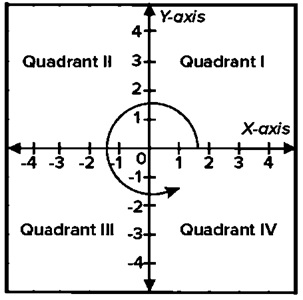Here, we are going to learn how to read coordinate points and determine its quadrant using C program?
Submitted by Nidhi, on August 11, 2021

Problem Solution:

There are four Quadrants based on the origin (Meeting point of the two axes).

1. In the first quadrant, both x and y have positive values.
2. In the second quadrant, x is negative and y is positive.
3. In the third quadrant, x and y are negative
4. In the fourth quadrant, x is positive and y is negative.

Consider the following graph,Program:

The source code to read coordinate points and determine their quadrant is given below. The given program is compiled and executed using GCC compile on UBUNTU 18.04 OS successfully.

```// C program to read coordinate points

#include <stdio.h>

{
if (X == 0 && Y == 0)
return 0;
else if (X > 0 && Y > 0)
return 1;
else if (X < 0 && Y > 0)
return 2;
else if (X < 0 && Y < 0)
return 3;
else if (X > 0 && Y < 0)
return 4;
return -1;
}

int main()
{
int X;
int Y;

int ret = 0;

printf("Enter the value of X: ");
scanf("%d", &X);

printf("Enter the value of Y: ");
scanf("%d", &Y);

if (ret == 0)
printf("Point (%d, %d) lies at the origin\n", X, Y);
else if (ret == 1)
printf("Point (%d, %d) lies in the First quadrant\n", X, Y);
else if (ret == 2)
printf("Point (%d, %d) lies in the Second quadrant\n", X, Y);
else if (ret == 3)
printf("Point (%d, %d) lies in the Third quadrant\n", X, Y);
else if (ret == 4)
printf("Point (%d, %d) lies in the Fourth quadrant\n", X, Y);

return 0;
}
```

Output:

```RUN 1:
Enter the value of X: 10
Enter the value of Y: 20
Point (10, 20) lies in the First quadrant

RUN 2:
Enter the value of X: -10
Enter the value of Y: 20
Point (-10, 20) lies in the Second quadrant

RUN 3:
Enter the value of X: -10
Enter the value of Y: -20
Point (-10, -20) lies in the Third quadrant

RUN 4:
Enter the value of X: 10
Enter the value of Y: -20
Point (10, -20) lies in the Fourth quadrant
```

Explanation:

In the above program, we created two functions determineQuadrant(), main(). The determineQuadrant() function is used to find the quadrant based on given coordinates.

In the main() function, we read the values of coordinates X and Y. Then we determined the quadrant based on the value of coordinates and printed the appropriate message on the console screen.